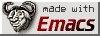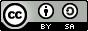# Some notes on bitwise art

... using GNU/Octave.
```-- bitxor (X, Y)
Return the bitwise XOR of non-negative integers X and Y.

-- bitand (X, Y)
Return the bitwise AND of non-negative integers.
X, Y must be in the range [0,intmax]

-- bitor (X, Y)
Return the bitwise OR of non-negative integers X and Y.

-- bitcmp (A, K)
Return the K-bit complement of integers in A.

If K is omitted 'k = log2 (flintmax) + 1' is assumed.

-- bitshift (A, K)
-- bitshift (A, K, N)
Return a K bit shift of N-digit unsigned integers in A.

A positive K leads to a left shift; A negative value to a right
shift.

If N is omitted it defaults to 64.  N must be in the range [1,64].
```